Capitalisation weighted indexes are considered to be representative of the broad market opportunity set and are characterised by high levels of liquidity, investment capacity and relatively low levels of turnover. However, concentration risks that may arise during market bubbles and the inherent factor traits of capitalisation weighted indexes have prompted alternative approaches to index construction, with the resulting indexes commonly referred to as “smart beta”. Smart beta indexes encompass both alternatively weighted and factor indexes.

Alternatively weighted indexes have a variety of objectives. For example, equally weighted indexes achieve increased diversification by allocating capital equally across constituents. Fundamental indexes weight stocks by their economic scale, resulting in a weighting scheme that is independent of price-based market measures of size. Risk based alternatively weighted indexes are typically based on a Markowitz optimisation, yet their objectives may be distinct: minimum variance indexes aim to achieve volatility reduction relative to the capitalisation weighted benchmark; equal risk contribution indexes are designed to improve risk diversification; and risk-efficient indexes aim to maximise the expected Sharpe Ratio.

An incidental outcome of alternative weighting approaches is a sometimes unintentional and/or uncontrolled exposure to risk factors. And while alternatively weighted indexes are intended to achieve certain objectives, they are also frequently used as a means of capturing the long-term risk premia associated with various factors. An alternative way of capturing factor risk premia is via diversified, transparent and replicable factor indexes, which are designed to exhibit controlled exposure to the factor or factors of interest.

In this report, we discuss ways of defining and calculating factor exposure. We demonstrate the factor exposures implicit in a range of alternatively weighted indexes and contrast them with the factor exposures of comparable factor indexes (i.e., indexes with explicit factor objectives). The results suggest that whilst alternatively weighted indexes do exhibit factor exposures, these exposures may be relatively weak, time-varying and are often diluted by exposure to non-target factors. We demonstrate that factor indexes, in a general framework, are better suited for capturing desired exposures than alternatively weighted indexes, particularly in the context of multiple factor objectives.

We also highlight the importance of taking a holistic approach when seeking multiple factor objectives. Simply aggregating single factor indexes may defeat the objective of achieving substantial exposure to multiple factors, since a combination of negatively correlated single factors can result in an undesired dilution of the intended factor exposures. For example, a simple combination of a value index and a quality index may result in relatively low exposure to both factors. We propose an alternative approach, which results in greater exposure to both factors. We demonstrate that an index with exposure to a particular factor (for example, value) can achieve additional factor objectives by tilting from the existing position towards those objectives (for example, towards quality). The resulting index preserves its original value focus while also offering exposure to quality.

1. Alternatively weighted indexes and factor Indexes

1.1 Alternatively weighted indexes

Alternatively weighted indexes were originally developed to address the concentration risks that may arise periodically in capitalisation weighted indexes. For example, during periods such as the technology, internet and telecommunications bubble of 1999-2000, capitalisation weighted indexes became highly concentrated in particular stocks and sectors.

The most intuitive alternative to a capitalisation weighted index is an equally weighted index. By comparison with capitalisation weighting, an equally weighted index achieves enhanced diversification by allocating equal amounts of capital to each index constituent. From a factor perspective, an equally weighted index displays a bias towards the size factor, since by comparison with a capitalization weighted index an equally weighted index allocates larger weightings to small capitalisation stocks (the factor exposures of alternatively weighted indexes are discussed at length in Section 3 of this paper).

Minimum variance indexes are constructed with the objective of minimising index risk by means of a mathematical optimisation, using stocks’ historical volatility and correlations as inputs. Unconstrained formulations of the minimum variance approach (e.g., Black et al., 1972) display high levels of concentration. Most index providers employ constraints in order to limit the degree of concentration in individual countries, sectors and stocks. In the FTSE Global Minimum Variance Index Series, FTSE incorporates an explicit diversification constraint to mitigate concentration concerns.

In an equal risk contribution (ERC) index, the diversification objective is achieved by recognising that equal weighting does not result in equal contributions to index level risk (since stocks have different volatilities and correlations). Instead, the exante contribution to index-level risk of each index constituent is equalised by means of an optimisation. Empirical studies (FTSE, 2013) illustrate that the ex-post risk contribution of each stock is also approximately equal. The concentration and volatility levels of an ERC index lie between those of an equally weighted and a minimum variance index. From a factor perspective, ERC indexes therefore exhibit both size and low volatility factor exposures.

Risk-efficient indexes (such as the FTSE EDHEC-Risk Efficient Index Series) aim to improve the capitalisation weighted index’s risk/return trade-off by positing a positive relationship between downside risk and expected return. The resulting optimisation results in an index with the highest expected return per unit of volatility (i.e., the maximum expected Sharpe Ratio).

Fundamental indexes use a company’s economic footprint (as opposed to market capitalisation) to determine constituent weightings. The FTSE RAFI Index Series use sales, cash flow, book value and dividends as measures of company size. Fundamental indexes share many desirable characteristics of capitalisation weighted indexes, such as relatively high levels of liquidity and investment capacity. The non-price-based weighting scheme suggests that they are less susceptible to bubbles or momentum-driven market excesses.

Equally weighted, risk based and fundamental indexes all attempt to overcome perceived drawbacks as compared to capitalisation weighted indexes. Table 1 summarises these alternatively weighted indexes and their objectives.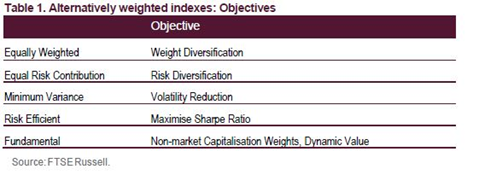An incidental outcome of alternative weighting approaches is exposure to common risk factors that have historically been associated with positive long run risk premia. While alternatively weighted indexes achieve their initial objectives, they are also frequently used as a means of capturing risk premia. However, the increasing interest in factors has resulted in the creation of diversified, transparent and replicable factor indexes, which are designed to exhibit controlled and explicit exposure to single or multiple factors.

1.2 Factor Indexes

Factor indexes have the specific objective of providing exposure to a particular factor or factors. For example, the FTSE Global Factor Index Series aims to achieve controlled exposure to specific stock-level characteristics, such as size, value and momentum (see FTSE, 2014a), with due consideration for the diversification and capacity characteristics of the resulting factor index.

To highlight the distinction between alternatively weighted and factor indexes, consider the characteristics of a minimum variance index (a popular alternative weighting methodology) and a low volatility factor index. While the minimum variance index has the objective of minimising volatility, the low volatility factor index aims to capture the factor premium associated with low volatility stocks, with relatively small incidental reductions in index-level volatility. The distinction between a FTSE Minimum Variance index and a FTSE Low Volatility Factor Index is discussed at greater length in Section 3.

The weights of a FTSE Factor Index are determined in the following manner. For stocks in an underlying index universe U, with underlying index weights Wi, the factor index weights are: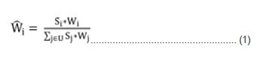Where Si is the standard cumulative normal distribution function of the crosssectional Z-Scores for a given factor.

Formula (1) amounts to the application of a tilt towards the factor of interest, where the strength and direction of the tilt may be varied (see FTSE 2014a for further information on the construction methodology for FTSE’s Global Factor Indexes).

The underlying index weights Wi may reflect any chosen weighting methodology (e.g., capitalisation, equal, or risk-based). However, a practical choice of underlying index in formula (1) is the capitalisation weighted index. Asness (2006), shows that when the underlying index is capitalisation weighted and S is the dividend yield, formula (1) results in an index that is similar to a fundamental index, constructed using dividends.

Exposure to multiple factors may be achieved by creating a composite of the factors of interest and the application of formula (1) or by the sequential or repeated application of a series of single factor tilts (i.e., the product of several Si). When applied to the underlying index weights, this sequential tilting results in an index that is tilted first towards one factor and then towards another. The result is that the final index exhibits both factor characteristics. Let S1i and S2i be the standard cumulative normal of cross-sectional Z-Scores of factors 1 and 2 of the ith stock. Then the weight of this stock in the twice tilted index is given by: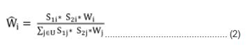In Section 2 we discuss approaches to assessing factor exposures. In Sections 3 and 4 we examine the factor exposures of selected alternatively weighted and factor indexes, highlighting the exposure to both target and to non-target (incidental) factors. In Section 5 we examine approaches to achieving simultaneous exposure to multiple factors.

2. Assessing factor exposure

The calculation of an index’s factor exposure requires the knowledge of stocks’ weightings through time.

Typically, raw factor scores are normalised by removing the sample mean and dividing by the sample standard deviation. The distribution of the resulting standardised factor scores (or “Z-Scores”) then has a mean of zero and a standard deviation of one.

The exposure of an index to a given factor may be derived as the aggregate of the individual stock weights, multiplied by the Z-Score of each stock. Since the factor score is standardised at each point in time, meaningful comparisons can be made regarding factor exposure through time. Let X be the exposure of an index to the

factor of interest, and Wj and Zj the index weight and the Z-Score of stock j with respect to the same factor: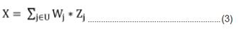Active factor exposure is defined as the difference between the index factor exposure and the underlying index factor exposure.

Factor exposure can also be assessed using a returns based analysis. Index excess returns are regressed over some period of time against the returns to a set of standard factor portfolios. The factor exposures, which we refer to as loadings, are then the beta regression coefficients. This is particularly useful approach when holdings based information is unavailable.

However there is an inherent ambiguity in this approach. First, what period of time should be used to make the assessment? Surely it should span a variety of market conditions so as not to bias results. However apart from “the longer the better” no consensus exists. More seriously what is the standard set of factors or factor portfolios? Should we use the Fama and French three factors (Fama and French (1992)), the Carhart four factors (Carhart (1997)) or factors derived from a set of factor mimicking portfolios (Grinold and Kahn (1995))?

The choice of factor definitions, portfolio construction technique and factors themselves will all affect the assessment of factor loadings.

Our view is that when holdings information is available exposure should be calculated as in equation (3). It is unambiguous and furthermore can be used to assess factor exposure at a point in time.

3. Factor exposure of alternatively weighted Indexes

In this section we follow the approach discussed in Section 2 to measure the factor exposures of a set of alternatively weighted indexes. Factor exposures are calculated using the factor definitions set out in the FTSE Global Factor Index Series Ground Rules (see FTSE, 2014e) and Appendix E.

3.1 Equally Weighted Indexes

In Table 2 we examine the average factor exposures of an equally weighted version of the FTSE Developed Index relative to the capitalisation weighted FTSE Developed Index from September 2001 through July 2015.

The size, volatility and illiquidity exposures of the equally weighted index are unsurprising. An equally weighted index is underweight large-cap stocks, leading to a positive exposure to size. Market capitalisation and the size factor are highly correlated with liquidity; consequently, a tilt towards small capitalisation stocks also manifests itself as exposure to relatively illiquid stocks. Furthermore, smaller stocks tend to have higher observed levels of volatility (i.e., a negative exposure to low volatility).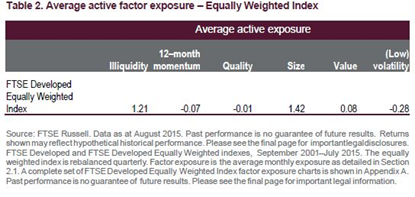3.2 Fundamental indexes

Table 3 exhibits the average factor exposure of the FTSE RAFI Developed 1000 Index11 relative to the FTSE Developed Index from March 2007 through July 2015. Fundamental company size is correlated with market capitalisation, leading to a negligible exposure to the illiquidity and size factors.

The value (and momentum) exposure of fundamental indexes is unsurprising. Asness (2006) provides one perspective of fundamental indexes as a value tilt on a capitalisation weighted index. Value stocks are frequently out of favour and consequently typically exhibit poor momentum. Quality stocks tend to be expensive; hence, a fundamentally weighted index will also display negative quality exposure.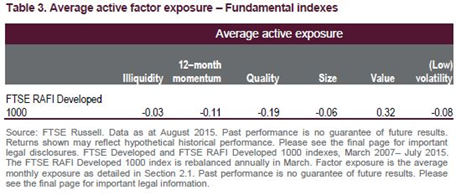The value and quality exposures of the fundamental index vary through time (see Figures 1 and 2). For example, as equity valuations became increasingly depressed during the 2008 financial crisis, the fundamental index became increasingly valueorientated (Figure 1). During the same period, the increased value exposure was juxtaposed with increasingly negative quality exposure (Figure 2).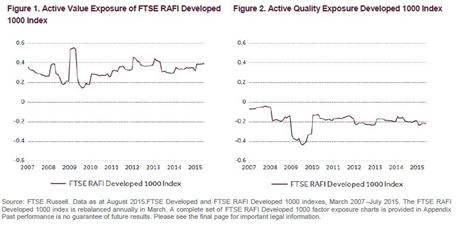3.3 Risk-Based Indexes

In Table 4 we show the average monthly active factor exposures of three risk-based indexes relative to the FTSE Developed Index from September 2003 through July 2015. Risk-based indexes (in particular the FTSE Developed Minimum Variance Index and FTSE EDHEC-Risk Efficient Developed Index) exhibit a substantial positive exposure to the size and illiquidity factors.

However, the risk-based indexes display variable exposure to the volatility factor: the FTSE Developed Minimum Variance Index in particular has a positive exposure to the low volatility factor, while the FTSE EDHEC-Risk Efficient Developed index exhibits a negative exposure (i.e., it is exposed to stocks exhibiting above-average levels of volatility). This is possibly the result of the index’s specification of expected return as a positive function of downside risk.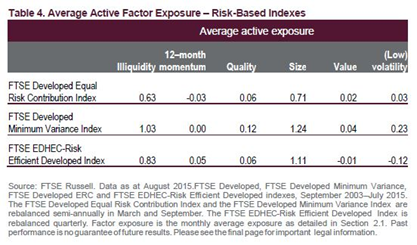In Figures 3-6 we show the individual active factor exposures of the three risk-based indexes over time. Risk-based indexes aim to achieve improved risk-adjusted outcomes and/or levels of diversification. The latter objective typically results in consistent positive exposure to size (see Figure 3). However, the value exposure of risk-based indexes varies considerably through time and is currently close to zero (Figure 4). In Figure 5, both the FTSE Developed Minimum Variance and FTSE Developed Equal Risk Contribution indexes exhibit a time varying low volatility tilt, a consequence of aiming to minimise total risk. Conversely, the FTSE EDHEC-Risk

Efficient Developed index is orientated towards higher (downside) volatility stocks. This finding is consistent with Blitz (2003). High-quality stocks typically display low levels of volatility and as a consequence both the FTSE Developed Minimum Variance and ERC indexes have (a small) positive exposure to quality (Figure 6).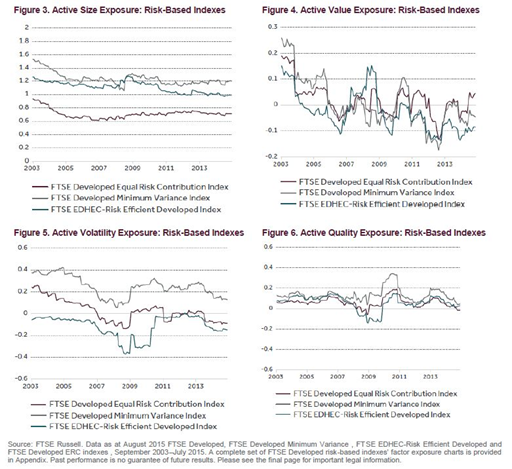In this section, we have shown that alternatively weighted indexes exhibit significant and variable exposures to common factors such as value, momentum, volatility and size. There is a significant body of work2 documenting the existence of long-run factor return premia attributable to these risk factors.

Alternatively weighted indexes are commonly combined in order to diversify model or weighting risk, and increasingly to achieve single and multiple factor objectives. In Sections 4 and 5 we show that factor exposure may also be achieved through dedicated factor indexes. Single factor indexes aim to provide exposure to a target risk factor whilst limiting exposure to non-target factors. We illustrate that the factor outcomes associated with single factor indexes are robust compared to an equivalent alternatively weighted index. In Section 5, we propose an alternative approach to constructing multiple factor indexes. We highlight that multiple factor objectives may be achieved more effectively through dedicated factor indexes than combining alternatively weighted indexes which may result in uncontrolled exposure to non-target factors and relatively weak exposure to the factors of interest.